# How are pictures created through refraction?

## Refraction¶

### Optical lenses¶

Cut glasses can bundle or scatter incident light rays - depending on the design. Such glass-like bodies, which are usually circular and have a certain curvature, are called "optical lenses". A well-known example is the magnifying glass, which can be used to obtain an enlarged image of an object.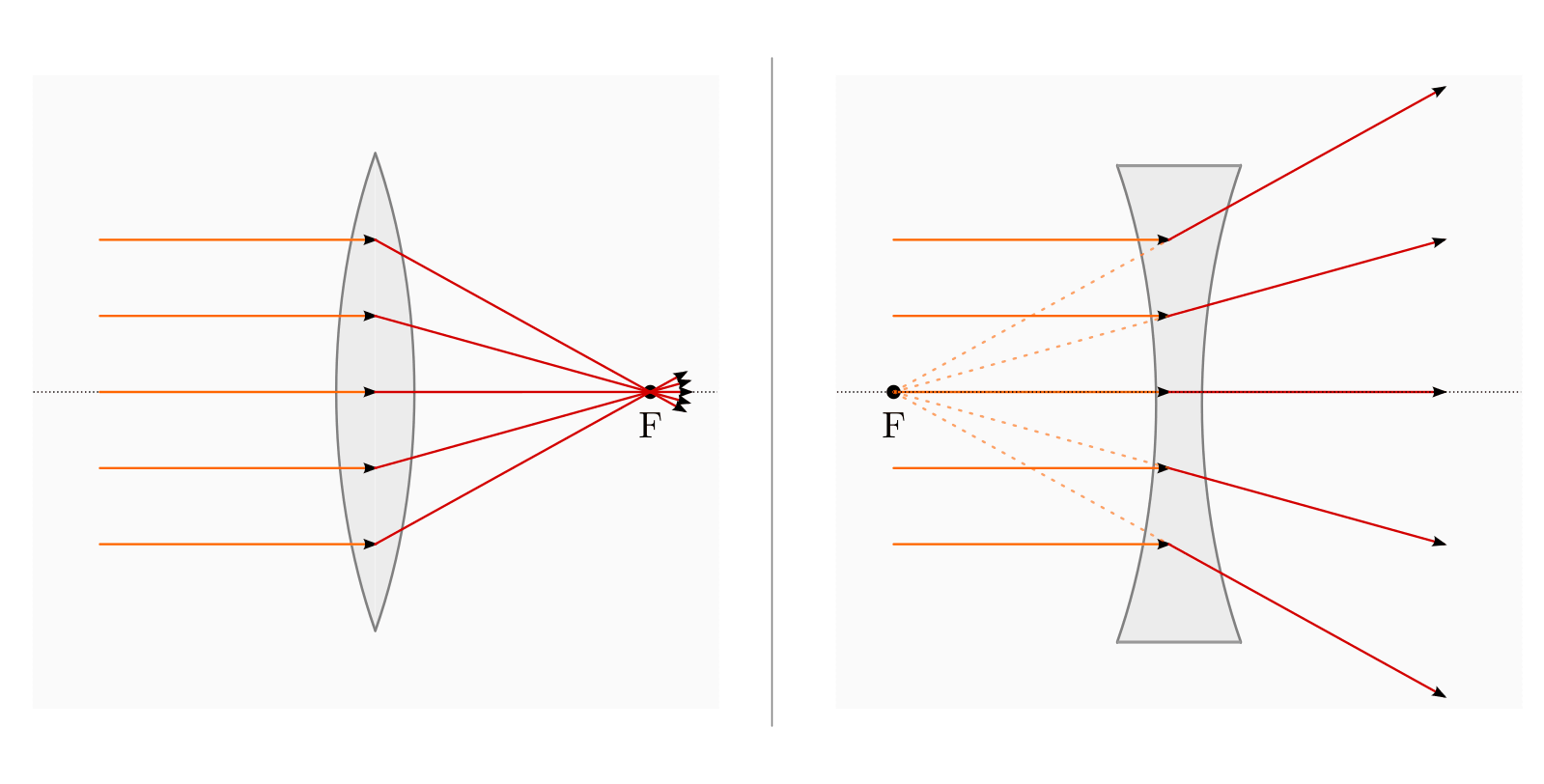Converging lens (left picture) and diverging lens (right picture) as typical shapes of optical lenses.

In order to explain the creation of an image on an optical lens, the same terms are used (as far as possible) as when describing curved mirrors:

• Optical axis:

The straight line that runs through the center of the lens and is perpendicular to the plane of the lens is called the optical axis.

• Parallel beams:

All rays that hit an optical lens parallel to the optical axis are called parallel rays. They are collected or scattered by the lens and intersect at a common point behind or in front of the lens. This point on the optical axis becomes the focal point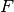called.

The distance between the center of the lens and a focal point becomes the focal length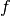called. The focal length of an optical lens is greater, the flatter the lens.

• Focus rays:

All rays that pass through a focal point of an optical lens are refracted by the lens in such a way that they run parallel to the optical axis behind the lens.

• Center rays:

Rays that pass through the center of the lens are refracted just as strongly when they enter the lens glass as they are when they exit. Central rays are therefore only shifted slightly in parallel (hardly noticeable with thin lenses). To a good approximation, center rays therefore pass through the lens unchanged.

One draws for any point of the object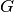two or three of the rays mentioned above, one obtains the appropriate image point as the intersection of the rays.

Image formation on a converging lens

When an object is shown through a converging lens, the position and size of the image depend on the distance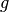the object to the lens and its focal lengthfrom.

If one approaches an object of a converging lens from a great distance, the image also moves away from the converging lens. Collecting lenses produce inverted, reversed images of objects when they are out of focus.

The focal point and parallel rays emanating from an object point, which are in turn imaged onto parallel or focal point rays by the converging lens, are sufficient for the construction of the image. The point of intersection of the refracted rays corresponds to the position of the image.

If one approaches an object from the focal point of a converging lens, the image also approaches the converging lens. Collecting lenses produce enlarged and upright images of the objects when they are within the focal length (magnifying glass effect).

To construct the image, the image rays emanating from an object point are drawn further behind the converging lens. It should be noted that focal point rays become parallel rays and central point rays always hit the lens perpendicularly and thus pass through it without refraction. The position of the image corresponds to the point of intersection of the elongated parallel or central rays behind the object.

Because of their magnifying effect, flat converging lenses (with a long focal length) are used, among other things, as magnifying glasses and lenses.

Image formation on a diverging lens

A diverging lens always produces upright, reduced images. If an object is brought closer to the lens surface, the image of the object also moves towards the lens and becomes larger in the process; however, it always remains smaller than the original.

To construct the image, a parallel and a focal ray are drawn in at a point on the object. The parallel beam is refracted by the diverging lens so that the rearward extension of the beam passes through the focal point. The (always reduced) image is located at the intersection of the parallel beam extended in this way with the central beam between the object and the lens.

Remarks: